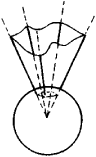# Polyhedral Angle

Also found in: Dictionary, Thesaurus.

## polyhedral angle

[¦päl·i¦hē·drəl ′aŋ·gəl]
(mathematics)
The shape formed by the lateral faces of a polyhedron which have a common vertex.
McGraw-Hill Dictionary of Scientific & Technical Terms, 6E, Copyright © 2003 by The McGraw-Hill Companies, Inc.
The following article is from The Great Soviet Encyclopedia (1979). It might be outdated or ideologically biased.

## Polyhedral Angle

a part of space bounded by one sheet of a polyhedral conical surface whose directrix is a plane polygon without self-intersections. The faces of the surface are the faces of the polyhedral angle, and the apex of the surface is the vertex of the polyhedral angle. A polyhedral angle is said to be regular if all its linear angles and all its dihedral angles are equal. The measure of a polyhedral angle is the area bounded by a spherical polygon (see Figure 1) formed by the intersection of the faces of the polyhedral angle by a sphere with a radius of 1 and a center at the vertex of the polyhedral angle.Figure 1